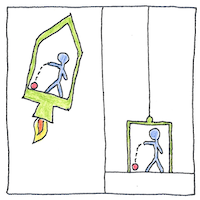# 1915 General relativity

## The book of science

Tom Sharp

 Albert Einstein physics## General relativity

• Einstein’s
• idea that
• spacetime is (as
• stated) curved by the
• energy or momentum of masses
• reconciles Newton’s law of universal gravitation—
• with its instantaneous attraction or action at
• a distance—with Einstein’s special relativity
• in which the speed of
• light is constant in
• all frames of
• reference.
• Let’s
• say
• mass
• or energy
• compresses or expands
• the framework of the
• universe and curvature means that
• the density of space
• increases and the
• density of
• time
• decreases.
• Nevertheless, the
• shape or density
• of spacetime affects the
• behavior of mass and energy—
• drawing planets to orbit the sun
• in ellipses warped by other planets, increasing
• the frequencies of electromagnetic waves, bending
• light around stars, and allowing
• massive objects to suck
• up everything around
• them, including
• light.

## Solutions

• Einstein assumed that his field equations were unsolvable.
• Eventually, Schwarzschild found a solution for a spherical spacetime;
• Friedmann and Lemaítre found solutions for expanding and contracting universes;
• Reissner and Nordström found a solution for spherical objects;
• and Kerr and Newman found a solution for massive rotating objects.
• The theory was generally regarded as true
• when maybe only three people in the world understood it.

## Moving

• Moving
• I deliberately set myself off balance.
• Moments
• I can tell simple things are misleading.
• Mondays
• all the rules have to be rewritten.
• Maybe
• even my wife doesn’t understand me.

The common two-dimensional illustration, in which the weight of a planet is shown depressing the fabric of a sheet of space, is misleading. A ball bearing rolling on the sheet and approaching the planet would be pulled by gravity into the depression, but—wait—a planet is not pulled down by gravity, and it attracts an object in space toward its center.

Light takes longer to travel through a gravitational field, I understand, because increasing gravity is the same as increasing the density of space. Increasing gravity dilates time and increases electromagnetic frequencies; decreasing gravity compresses time and decreases electromagnetic frequencies.

Why this happens, why the shape or density of spacetime explains what we experience as gravity, to me, is still a mystery, unless gravitational acceleration occurs simply as a means of conserving energy and the weights we feel are inertial.

See also in The book of science: